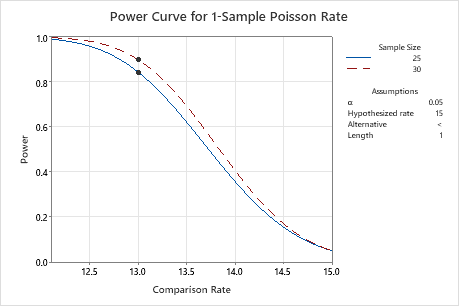# Interpret the key results for Power and Sample Size for 1-Sample Poisson Rate

Complete the following steps to interpret Power and Sample Size for 1-Sample Poisson Rate. Key output includes the comparison rate, the sample size, the power, and the power curve.

## Step 1: Examine the calculated values

Using the values of the two power function variables that you entered, Minitab calculates the comparison rate, the sample size, or the power of the test.

Comparison rate

Minitab calculates the comparison rate. The difference between the comparison rate and the hypothesized rate is the minimum difference for which you can achieve the specified level of power for each sample size. Larger sample sizes enable the test to detect smaller differences. You want to detect the smallest difference that has practical consequences for your application.

Sample size

Minitab calculates how large your sample must be for a test with your specified power to detect the difference between the hypothesized rate and comparison rate. Because sample sizes are whole numbers, the actual power of the test might be slightly greater than the power value that you specify.

If you increase the sample size, the power of the test also increases. You want enough observations in your sample to achieve adequate power. But you don't want a sample size so large that you waste time and money on unnecessary sampling or detect unimportant differences to be statistically significant.

Power

Minitab calculates the power of the test based on the specified comparison rate and sample size. A power value of 0.9 is usually considered adequate. A value of 0.9 indicates you have a 90% chance of detecting a difference between the hypothesized rate and the population comparison rate when a difference actually exists. If a test has low power, you might fail to detect a difference and mistakenly conclude that none exists. Usually, when the sample size is smaller or the difference is smaller, the test has less power to detect a difference.

## Step 2: Examine the power curve

Use the power curve to assess the appropriate sample size or power for your test.

The power curve represents every combination of power and comparison rate for each sample size when the significance level is held constant. Each symbol on the power curve represents a calculated value based on the values that you enter. For example, if you enter a sample size and a power value, Minitab calculates the corresponding comparison proportion and displays the calculated value on the graph.

Examine the values on the curve to determine the difference between the comparison rate and the hypothesized rate that can be detected at a certain power value and sample size. A power value of 0.9 is usually considered adequate. However, some practitioners consider a power value of 0.8 to be adequate. If a hypothesis test has low power, you might fail to detect a difference that is practically significant. If you increase the sample size, the power of the test also increases. You want enough observations in your sample to achieve adequate power. But you don't want a sample size so large that you waste time and money on unnecessary sampling or detect unimportant differences to be statistically significant. If you decrease the size of the difference that you want to detect, the power also decreases.

In this graph, the power curve for a sample size of 25 shows that the test has a power of approximately 0.84 for a comparison rate of 13. For a sample size of 30, the power curve shows that the test has a power of approximately 0.9 for a comparison rate of 13. As the comparison rate approaches the hypothesized rate (15 in this graph), the power of the test decreases and approaches α (also called the significance level), which is 0.05 for this analysis.By using this site you agree to the use of cookies for analytics and personalized content.  Read our policy